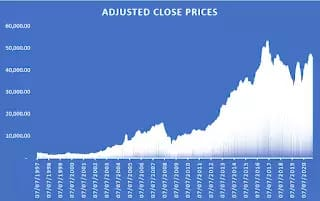## Assumptions of Classical Linear Regression Model-CLRM-Based on secondary data

Assumptions of the Classical Linear Regression Model Introduction: To forecast the value of a dependent variable based on the values of one or more independent variables, statisticians utilize the Classical Linear Regression Model (CLRM) based on “Assumptions of the Classical Linear Regression Model”. It is one of the statistical models that is employed the most …